Search IntMath
Close

# Converting Complex Numbers

## Choosing a Calculator

The first example is applicable to most advanced calculators, while the second example is specific to Casio calculators. If you need help determining which calculator is best for you, see our review guide here: How to Buy a Calculator.

You can find our review of the Casio FX: Casio FX Calculator Review.

### Example 1

Convert 56\ ∠\ 27^@ to rectangular form (x + jy), where j=sqrt(-1).

 Keystrokes Display 56 56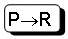56 27 27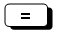49.89636535 (= x)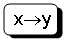25.42346799 (= y)

Therefore, 56\ ∠\ 27^@ ≈ 49.9 + 25.4 j

We have converted a complex number from polar form (using degrees) into rectangular form.

Of course, you have to be careful that you have your calculator set correctly in degrees (or radians, if required).

### Example 2

Convert 12 - 42j to polar form (r ∠ theta).

For CASIO calculator...

 Keystrokes Display 12 12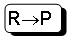12 42 42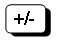-4243.68065934 (= r)-74.0546041 (= θ)

Therefore:

Complex number in rectangular form: 12 - 42j

Same complex number in polar form: 43.7 ∠ -74^@

For Sharp calculator, it is a similar process.

Consult your calculator manual for full details.

## Problem SolverThis tool combines the power of mathematical computation engine that excels at solving mathematical formulas with the power of GPT large language models to parse and generate natural language. This creates math problem solver thats more accurate than ChatGPT, more flexible than a calculator, and faster answers than a human tutor. Learn More.